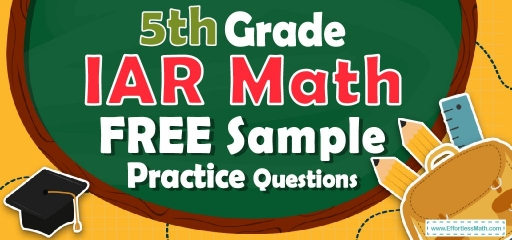# 5th Grade IAR Math FREE Sample Practice QuestionsWhat measures have you taken to prepare your 5th-grade student as much as possible to take the IAR math test? To pass the Illinois Assessment of Readiness (IAR) math test, a 5th-grade student will need to use a sample of practice questions to prepare better for the IAR math test. This helps a lot to calm and increase the students’ self-confidence during the IAR math test.

Here are brief but valuable Free IAR math sample practice questions to help 5th-grade students.

There are many benefits to using these sample practice questions for 5th-grade students, including being able to identify and address their weaknesses.

These sample questions have been prepared by the most up-to-date research team to prepare your 5th-grade students for the IAR 2022 math test.

Be sure to refer to the related links at the bottom of this post to give you a better understanding of what your 5th-grade student needs to take the IAR math test.

The Absolute Best Book to Ace the 5th Grade IAR Math Test

## 10 Sample 5th Grade IAR Math Practice Questions

1- What is the volume of this box?
$$\img {https://appmanager.effortlessmath.com/public/images/questions/test5.iar5.222.JPG }$$

A. $$24 cm$$$$^3$$

B. $$32 cm$$$$^3$$

C. $$162 cm$$$$^3$$

D. $$192 cm$$$$^3$$

2- How many square feet of tile is needed for $$18$$ feet to $$18$$ feet room?

A. $$72$$ Square Feet

B. $$108$$ Square Feet

C. $$216$$ Square Feet

D. $$324$$ Square Feet

3- Of the $$2,400$$ videos available for rent at a certain video store, $$600$$ are comedies. What percent of the videos are comedies?

A. $$18 ½ \%$$

B. $$20\%$$

C. $$22\%$$

D. $$25\%$$

4- How many $$3 × 3$$ squares can fit inside a rectangle with a height of $$54$$ and width of $$12$$?

A. $$72$$

B. $$62$$

C. $$50$$

D. $$44$$

5- ABC Corporation earned only $$200,000$$ during the previous year, two-thirds only of the management’s predicted income. How much earnings did the management predict?

A. $$20,000$$

B. $$30,000$$

C. $$300,000$$

D. $$340,000$$

6- William keeps track of the length of each fish that he catches. Following are the lengths in inches of the fish that he caught one day: $$13, 14, 9, 11, 9, 10, 18$$
What is the median fish length that William caught that day?

A. $$11$$ Inches

B. $$9$$ Inches

C. $$12$$ Inches

D. $$13$$ Inches

7- Solve: $$9 + [8 × 5] ÷ 2 =$$ ? ________

8- What is the median of these numbers? $$4, 9, 13, 8, 15, 18, 5$$

A. $$8$$

B. $$9$$

C. $$13$$

D. $$15$$

9- The area of the base of the following cylinder is $$50$$ square inches and its height is $$10$$ inches. What is the volume of the cylinder? ________

10- A rope $$13$$ yards long is cut into $$4$$ equal parts. Which expression does NOT equal the length of each part?

A. $$13 ÷ 4$$

B. $$\frac{13}{4}$$

C. $$4 ÷ 13$$

D. $$4\sqrt{13}$$

Best 5th Grade IAR  Math Exercise Resource

1- D
Use the volume of the cube formula.
Voluem$$=$$ length $$×$$ width$$×$$ height$$⇒ V= 6 × 4 × 8 ⇒ V=192 \space$$$$cm^3$$

2- D
Find the area of the room which is a square. Use the area of the square formula.
$$S= a^2 ⇒ S= 18$$ feet $$× 18$$ feet $$= 324$$ square feet

3- D
Use the percent formula:
part$$=\frac{percent}{100}×$$whole
$$600 =\frac{percent}{100}× 2400⇒ 600=$$ percent $$× 24 ⇒$$percent$$=25$$

4- A
Use the area of the rectangle formula.
$$A= a × b ⇒ A= 54 × 12 ⇒ A= 648$$
Divide the area by $$9$$ ($$3 × 3 = 9$$ squares) to find the number of squares needed. $$648 ÷ 9 = 72$$

5- C
ABC Corporation’s income $$= \frac{2}{3}$$ management’s predicted income.
$$200,000 = \frac{2}{3}$$ management’s predicted income
management’s predicted income $$= 200,000×\frac{3}{2} =300,000$$

6- A
Write the numbers in order:
$$9, 9, 10, 11, 13, 14, 18$$
The median is the number in the middle. Therefore, the median is $$11$$.

7- 29
Use PEMDAS (order of operation):
$$9 + [8 × 5] ÷ 2 = 9 + (40) ÷ 2 = 9 + (40 ÷ 2) = 29$$

8- B
Write the numbers in order:
$$4, 5, 8, 9, 13, 15, 18$$
The median is the number in the middle. Therefore, the median is $$9$$.

9- 500
Use the volume of cylinder formula.
Voluem$$=$$ base $$×$$ height $$⇒ V=50 × 10 ⇒ V=500$$

10- C
$$13$$ yards long rope is cut into $$4$$ equal parts. Therefore, $$13$$ should be divided by $$4$$. Only option C is NOT $$13$$ divided by $$4$$. (It is $$4$$ divided by $$13$$)

The Best Books to Ace the 5th Grade IAR Math Test

### What people say about "5th Grade IAR Math FREE Sample Practice Questions - Effortless Math: We Help Students Learn to LOVE Mathematics"?

No one replied yet.

X
30% OFF

Limited time only!

Save Over 30%

SAVE $5 It was$16.99 now it is \$11.99